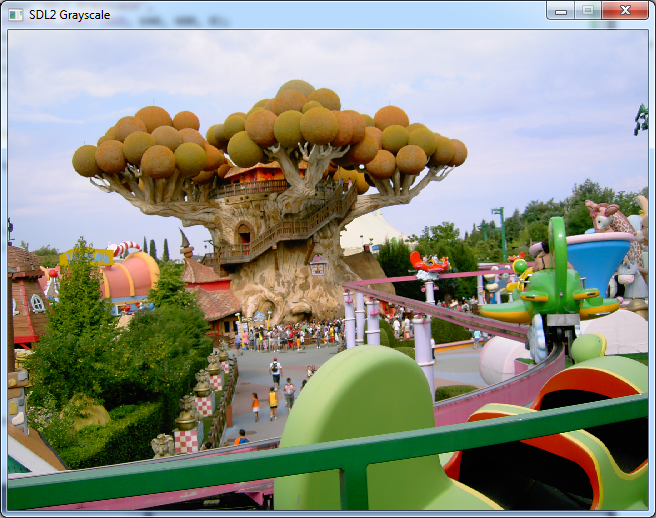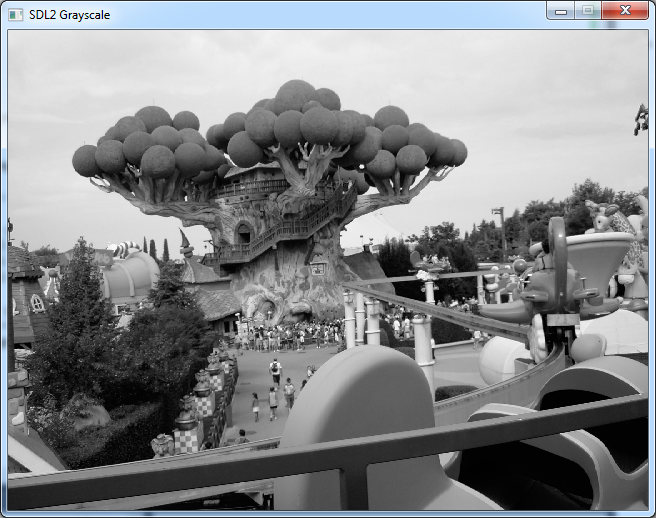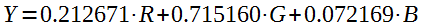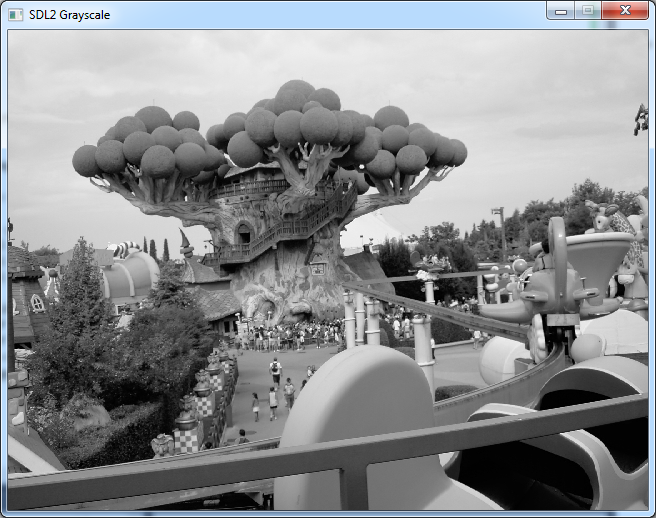# Converting an Image to Grayscale using SDL2

This article was originally posted on 22nd February 2014 at Programmer’s Ranch. It has been slightly updated here. The source code is available at the Gigi Labs BitBucket repository.

In the previous article, “SDL2 Pixel Drawing“, we saw how to draw pixels onto a blank texture that we created in code. Today, on the other hand, we’ll see how we can manipulate pixels on an existing image, such as a photo we loaded from disk. We’ll also learn how to manipulate individual bits in an integer using what are called bitwise operators, and ultimately we’ll convert an image to grayscale.

The first thing we’re going to do is load an image from disk. Fortunately, we’ve covered that already in “Loading Images in SDL2 with SDL_image“, so refer back to it to set things up. We’ll also start off with the code from the article, which, adapted a little bit, is this:

```#include <SDL.h>
#include <SDL_image.h>

int main(int argc, char ** argv)
{
bool quit = false;
SDL_Event event;

SDL_Init(SDL_INIT_VIDEO);
IMG_Init(IMG_INIT_JPG);

SDL_Window * window = SDL_CreateWindow("SDL2 Grayscale",
SDL_WINDOWPOS_UNDEFINED, SDL_WINDOWPOS_UNDEFINED, 640, 480, 0);
SDL_Renderer * renderer = SDL_CreateRenderer(window, -1, 0);
SDL_Texture * texture = SDL_CreateTextureFromSurface(renderer,
image);

while (!quit)
{
SDL_WaitEvent(&event);

switch (event.type)
{
case SDL_QUIT:
quit = true;
break;
}

SDL_RenderCopy(renderer, texture, NULL, NULL);
SDL_RenderPresent(renderer);
}

SDL_DestroyTexture(texture);
SDL_FreeSurface(image);
SDL_DestroyRenderer(renderer);
SDL_DestroyWindow(window);
IMG_Quit();
SDL_Quit();

return 0;
}
```

You see, the problem here is that we can’t quite touch the texture pixels directly. So instead, we need to work a little bit similarly to “SDL2 Pixel Drawing“: we create our own texture, and then copy the surface pixels over to it. So we throw out the line calling SDL_CreateTextureFromSurface(), and replace it with the following:

```    SDL_Texture * texture = SDL_CreateTexture(renderer,
SDL_PIXELFORMAT_ARGB8888, SDL_TEXTUREACCESS_STATIC,
image->w, image->h);
```

Then, at the beginning of the `while` loop, add this:

```        SDL_UpdateTexture(texture, NULL, image->pixels,
image->w * sizeof(Uint32));
```

If you try and run program now, it will pretty much explode. That’s because our code is assuming that our image uses 4 bytes per pixel (ARGB – see “SDL2 Pixel Drawing“). That’s something that depends on the image, and this particular JPG image is most likely 3 bytes per pixel. I don’t know much about the JPG format, but I’m certain that it doesn’t support transparency, so the alpha channel is out.

The good news is that it’s possible to convert the surface into one that has a familiar pixel format. To do this, we use SDL_ConvertSurfaceFormat(). Add the following before the `while` loop:

```    SDL_Surface * originalImage = image;
image = SDL_ConvertSurfaceFormat(image, SDL_PIXELFORMAT_ARGB8888, 0);
SDL_FreeSurface(originalImage);
```

What this does is take our surface (in this case the one that `image` points to) and return an equivalent surface with the pixel format we specify. Now that the new `image` has the familiar ARGB format, we can easily access and manipulate the pixels. Add the following after the line you just added (before the `while` loop) to typecast the surface pixels from `void *` to `Uint32 *` which we can work with:

```    Uint32 * pixels = (Uint32 *)image->pixels;
```

So far so good:Now, let’s add some code do our grayscale conversion. We’re going to convert the image to grayscale when the user presses the ‘G’ key, so let us first add some code within the `switch` statement to handle that:

```        case SDL_KEYDOWN:
switch (event.key.keysym.sym)
{
case SDLK_g:
for (int y = 0; y < image->h; y++)
{
for (int x = 0; x < image->w; x++)
{
Uint32 pixel = pixels[y * image->w + x];
// TODO convert pixel to grayscale here
}
}
break;
}
break;
```

This is where bit manipulation comes in. You see, each pixel is a 32-bit integer which in concept looks something like this (actual values are invented, just for illustration):

 Alpha Red Green Blue 11111111 10110101 10101000 01101111

So let’s say we want to extract the Red component. Its value is 10110101 in binary, or 181 in decimal. But since it’s in the third byte from right, its value is much greater than that. So we first shift the bits to the right by 16 spaces to move it to the first byte from right:

 Alpha Red 00000000 00000000 11111111 10110101

…but we still can’t interpret the integer as just red, since the alpha value is still there. We want to extract just that last byte. To do that, we perform a bitwise AND operation:

We do an AND operation between our pixel value and a value where only the last byte worth of bits are set to 1. That allows us to extract our red value.

In code, this is how it works:

```                            Uint8 r = pixel >> 16 & 0xFF;
Uint8 g = pixel >> 8 & 0xFF;
Uint8 b = pixel & 0xFF;
```

The `>>` operator shifts bits to the right, and the `&` is a bitwise AND operator. Each colour byte is shifted to the last byte and then ANDed with the value 0xFF, which is hexadecimal notation for what would be 255 in decimal, or 11111111 in binary. That way, we can extract all three colours individually.

We can finally perform the actual grayscaling operation. A simple way to do this might be to average the three colours and set each component to that average:

```                            Uint8 v = (r + g + b) / 3;
```

Then, we pack the individual colour bytes back into a 32-bit integer. We follow the opposite method that we used to extract them in the first place: they are each already at the last byte, so all we need to do is left-shift them into position. Once that is done, we replace the actual pixel in the surface with the grayscaled one:

```                            pixel = (0xFF << 24) | (v << 16) | (v << 8) | v;
pixels[y * image->w + x] = pixel;
```

If we now run the program and press the ‘G’ key, this is what we get:It looks right, doesn’t it? Well, it’s not. There’s an actual formula for calculating the correct grayscale value (`v` in our code), which according to Real-Time Rendering is:The origin of this formula is beyond the scope of this article, but it’s due to the fact that humans are sensitive to different colours in different ways – in fact there is a particular affinity to green, hence why it is allocated the greatest portion of the pixel colour. So now all we have to do is replace the declaration of `v` with the following:

```                            Uint8 v = 0.212671f * r + 0.715160f * g + 0.072169f * b;
```

And with this, the image appears somewhat different:This approach gives us a more even distribution of grey shades – in particular certain areas such as the trees are much lighter and we can make out the details more easily.

That’s all, folks! 🙂 In this article, we learned how to convert an image to grayscale by working on each individual pixel. To do this, we had to resort to converting an image surface to a pixel format we could work with, and then copy the pixels over to a texture for display in the window. To actually perform the grayscale conversion, we learned about bitwise operators which assisted us in dealing with the individual colours. Finally, although averaging the colour channels gives us something in terms of shades of grey, there is a formula that is used for proper grayscale conversion.

## One thought on “Converting an Image to Grayscale using SDL2”

1.Cuzin-Rambaud says:

thanks, good tuto 🙂